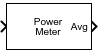Power Meter

Measure power of voltage signal

• Library:
• DSP System Toolbox / Statistics

•Description

The Power Meter block computes the power measurements of a voltage signal. The power measurements include average power, peak power, and peak-to-average power ratio. The block uses the sliding window method to compute these measurements. For more details, see Algorithms.

Ports

Input

expand all

Specify the input signal in volts as a vector or a matrix. If x is a matrix, each column is treated as an independent channel. The power measurement is computed along each channel using the Sliding Window Method.

Data Types: single | double
Complex Number Support: Yes

Output

expand all

Average power of the voltage signal, returned as a vector or a matrix, and measured in the units determined by the Power units parameter. For details on how the block computes the average power, see Average Power.

Dependencies

To enable this port, set Measurement to Average power or All.

Data Types: single | double

Peak power of the voltage signal, returned as a vector or a matrix, and measured in the units determined by the Power units parameter. For details on how the block computes the peak power, see Peak Power.

Dependencies

To enable this port, set Measurement to Peak power or All.

Data Types: single | double

Peak-to-average power ratio of the voltage signal, returned as a vector or a matrix. For details on how the block computes the peak-to-average power ratio, see Peak-to-Average Power Ratio.

Dependencies

To enable this port, set Measurement to Peak-to-average power ratio or All.

Data Types: single | double

Parameters

expand all

Specify the desired power measurement as one of the following:

• Average power (default)

• Peak power

• Peak-to-average power ratio

• All

For details on how the block computes these measurements, see Algorithms.

Specify the window length over which the measurement is computed as a positive integer.

Specify the reference load that the power meter uses to compute power values as a real, positive scalar in ovohms.

Tunable: Yes

Specify the units of the measured power values as one of the following:

• dBm

• dBW

• Watts

• Code generation: Simulate model using generated C code. The first time you run a simulation, Simulink® generates C code for the block. The C code is reused for subsequent simulations, as long as the model does not change. This option requires additional startup time but provides faster simulation speed than Interpreted execution.

• Interpreted execution: Simulate model using the MATLAB®  interpreter. This option shortens startup time but has slower simulation speed than Code generation.

Block Characteristics

 Data Types double | single Direct Feedthrough no Multidimensional Signals no Variable-Size Signals yes Zero-Crossing Detection no

expand all

Extended Capabilities

Blocks

Introduced in R2021a

DSP System Toolbox DocumentationGet trial now